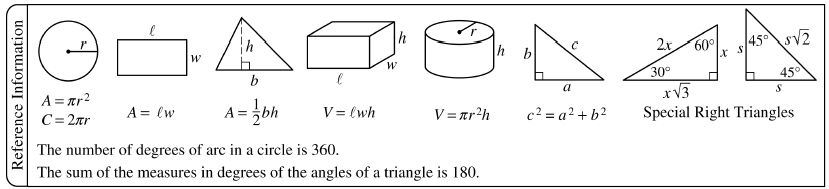Extra Hard SAT Math Question – FOIL or Factor?

At the end of every SAT Math section, the test makers try to come up with an extremely difficult problem that will leave even the cleverest studentsExtra Hard SAT Math Question – Speed Trap

At the end of every SAT Math section, the test makers try to come up with an extremely difficult problem that will leave even the cleverest students scratching theirExtra Hard SAT Math Question – Too Big for the Calculator

At the end of every SAT Math section, the test makers try to come up with an extremely difficult problem that will leave even the cleverest students scratching their heads. The reallyExtra Hard SAT Math Question – Prime Factorization

At the end of every SAT Math section, the test makers try to come up with an extremely difficult problem that will leave even the cleverest students scratching theirExtra Hard SAT Math Question – Finding the Sum of Way Too Many Numbers

At the end of every SAT Math section, the test makers try to come up with an extremely difficult problem that will leave even the cleverest students scratching their heads. The really evilThe easiest way to improve your SAT Critical Reading Score is to practice! This means going through example problem after example problem until you begin to memorize key vocabulary words and recognize the ways SAT test makers attempt to trickExtra Hard SAT Math Question – Way Too Many Variables

At the end of every SAT Math section, the test makers try to come up with an extremely difficult problem that will leave even the cleverest students scratching theirSAT Math Word Problem

The SAT is a Critical Reasoning test; this means that every question on the SAT will contain some element of Critical Reasoning to it. This can be particularly frustrating on the SAT Math section. For example,Know the SAT Math Formula (part 2 of 8)Today we continue our series on the SAT Math Formula sheet. To keep things interesting, today’s example problem is about Spencer, a super secret spy!Super Secret Spy Spencer wants to mow lawns and gather some quick intel. He